• ## C语言指针知识点总结

万次阅读 多人点赞 2020-05-11 09:37:26
以前总是搞不懂指针这一章节的知识，学得非常的混乱，这可不，马上要考试了，必须火力全开呀，今天在CSDN博客上看到一篇关于指针知识点总结，觉得受益匪浅，感触颇丰！ 指针 指针的定义： 指针是一个变量，用来...
以前总是搞不懂指针这一章节的知识，学得非常的混乱，这可不，马上要考试了，必须火力全开呀，今天在CSDN博客上看到一篇关于指针的知识点总结，觉得受益匪浅，感触颇丰！
指针
指针的定义： 指针是一个变量，用来存放地址的变量
指针的类型：
（1）基本类型：int 、short、long、float 、double、char 如 int *p；//表示定义一个指针，这个指针指向整形变量，这个指针将存放的是整形变量的地址。
**（2）数组指针：**指针指向一个数组 int （*a）;//表示指针指向一个int型的长度为10的数组
**（3）函数指针：**指针指向一个函数 void （*a）（）；//表示指针指向一个无参数无返回值的函数
指针的引用：指针引用后得到的是该地址所对应的变量的值。
二级指针：指向一级指针地址的指针
指针的运算： （1）指针+或者-一个数，表示指针加减这个数的类型所占内存的整数倍 如 int *p=a; p=p+2; // 在VC6.0里面，实际上p加了2 * 4=8
（2）指针减去一个指针，表示两个指针之间所差的内存单元或者元素个数，两个指针相加没有意义
（3）关系运算，如果定义了两个指针变量p和q，并且都已经初始化了， 如果p==q，则表示p和q指向同一个地址 p>q,则表示p指向高地址而q指向低地址 也经常拿p和NULL做比较，用来表示指针当前的状态，这在数据结构中应用非常广泛，不如说链表的查找等等
指针和数组的关系： 指针和数组关系密切，数组可以通过指针去引用，数组可以存放指针，数组也可以由指针去访问，指针也能存放数组的地址展开全文• ## C语言指针知识点小结

千次阅读 多人点赞 2020-01-10 15:40:06
C语言指针基础知识点(一)–指针及指针变量   C语言指针基础知识点(二)–指针变量的引用   C语言指针基础知识点(三)–指针变量作为函数参数   C语言指针基础知识点(四)–通过指针引用数组   C语言指针...
 前期回顾
C语言指针基础知识点(一)–指针及指针变量   C语言指针基础知识点(二)–指针变量的引用   C语言指针基础知识点(三)–指针变量作为函数参数   C语言指针基础知识点(四)–通过指针引用数组   C语言指针基础知识点(五)–用数组名作函数参数   C语言指针基础知识点(六)–通过指针引用多维数组   C语言指针基础知识点(七)–通过指针引用字符串   C语言指针基础知识点(八)–返回指针值的函数   C语言指针基础知识点(九)–指针数组和多重指针   C语言指针基础知识点(十)–动态内存分配与指向它的指针变量
指针小结
1. 指针的含义
指针就是地址，凡是出现"指针"的地方，都可以用"地址"代替，例如，变量的指针就是变量的地址，指针变量就是地址变量。
要区分指针和指针变量。指针就是地址本身，例如2008是某一变量的地址，2008就是变量的指针。而指针变量是用来存放地址的变量。指针变量的值是一个地址。
2. 什么叫"指向"
地址就意味着指向，因为通过地址能找到具有该地址的对象。对于指针变量来说，把谁的地址存放在指针变量中，就说此指针变量指向谁。但应注意：并不是任何类型数据的地址都可以存放在同一个指针变量中的，只有与指针变量的基类型相同的数据的地址才能存放在相应的指针变量中。例如：
int a, *p;  // p是 int * 型的指针变量，基类型是 int 型
float b;
p=&a;   // a 是 int 型，合法
p=&b;  // b 是 float 型，类型不匹配

既然许多数据对象(如变量、数组、字符串、函数等)都在内存中被分配存储空间，就有了地址，也就有了指针。可以定义一些指针变量，存放这些数据对象的地址，即指向这些对象。
void * 指针是一种特殊的指针，不指向任何类型的数据，如果需要用此地址指向某类型的数据，应先对地址进行类型转换。显示或隐示的类型转换。
3. 在对数组的操作中正确地使用指针
一维数组名代表数组首元素的地址，如：
int *p, a;
p=a;

p是指向 int 型类型的指针变量，显然，p 只能指向 a 数组中的元素，而不是指向整个数组。在进行赋值时一定要先确定赋值号两侧的类型是否相同，是否允许赋值。
对p = a;, 准确地说应该是：p 指向 a 数组的首元素，在不引起误解的情况下，有时也简称为：p指向a 数组。 同理，p 指向字符串，应理解为 p 指向字符串中的首字符
4. 指针变量的归纳比较
变量定义类型表示含义int *pint *定义指针数组p，它由4个指向整型数据的指针元素组成int (*p)int (*)p为指向包含4个元素的一维数组的指针变量int *p()int *()p为返回一个指针的函数，该指针指向整型数据int (*p)()int (*)()p为指向函数的指针，该函数返回一个整型值int **pint **p是一个指针变量，它指向一个指向整型数据的指针变量void *pvoid *p是一个指针变量，其基类型为 void，不指向具体的对象
5. 指针运算
a. 指针变量加减一个整数
例如： p++, p- -, p+i, p-i, p+=i, p-=i 等均是指针变量加(减)一个整数。   将该指针变量的原值和它指向的变量所占用的存储单元的字节数相加(减)
b. 指针变量赋值
将一个变量地址赋给一个指针变量。如：
p=&a;         //  将变量a的地址赋值给p
p=array;      //  将数组array首元素的地址赋给p
p=&array[i];  // 将数组 array 第 i 个元素的地址赋给p
p=max();      // max为已定义的函数，将max的入口地址赋给p
pa=p2;        // p1和p2是基类型相同的指针变量，将p2的值赋给p1

c. 两个指针变量可以相减
如果两个指针变量都指向同一个数组中的元素，则两个指针变量值之差是两个指针之间的元素个数。
d. 两个指针变量比较
若两个指针变量指向同一个数组中的元素，则可以进行比较。指向前面的元素的指针变量"小于"指向后面元素的指针变量。如果 p1 和 p2 不指向同一数组则比较无意义。
6. 指针变量可以有空值
指针变量可以不指向任何变量，可以这样表示：
p=NULL;

其中，NULL是一个符号常量，代表整数0。在stdio.h头文件中对NULL进行了定义：
# define NULL 0

它使p指向地址为0的单元。系统保证使该单元不作他用。
注意：
不应把一个整数赋给指针变量p的值为 NULL 与未对 p 赋值是两个不同的概念。任何指针变量或地址都可以与NULL作相等或不相等的比较，例如： if (p==NULL)
展开全文• 一、对星号 * 的总结 在我们目前所学到的语法中，星号主要有三种用途： 表示乘法，例如int a = 3, b = 5, c; c = a * b;，这是最容易理解的。 表示定义一个指针变量，以和普通变量区分开，例如int a = 100; int *p ...


本文目录
一、对星号 * 的总结二、各种指针总结1、数组指针2、字符串指针3、指针变量做函数参数4、指针作为函数返回值5、指针数组6、二维数组指针7、函数指针
三、指针对比四、空指针NULL与NUL以及void指针

一、对星号 * 的总结
在我们目前所学到的语法中，星号主要有三种用途：
表示乘法，例如int a = 3, b = 5, c; c = a * b;，这是最容易理解的。表示定义一个指针变量，以和普通变量区分开，例如int a = 100; int *p = &a;。表示获取指针指向的数据，是一种间接操作，例如int a, b, *p = &a; *p = 100; b = *p;。
二、各种指针总结
1、数组指针
如果一个指针指向了数组，我们就称它为数组指针（Array Pointer）
重点： 数组名是常量，它的值不能改变，而数组指针是变量（除非特别指明它是常量），它的值可以任意改变。也就是说，数组名只能指向数组的开头，而数组指针可以先指向数组开头，再指向其他元素。
2、字符串指针
表示方法
第一种方法直接使用字符数组表示字符串，声明一个指针指向字符数组的首地址：
char str[] = "http://c.biancheng.net";
char *pstr = str;

第二种方法是直接使用一个指针指向字符串（也称字符串常量）：
char *str = "http://c.biancheng.net";

两种表示方法的区别：
它们最根本的区别是在内存中的存储区域不一样，字符数组存储在全局数据区或栈区，第二种形式的字符串存储在常量区。全局数据区和栈区的字符串（也包括其他数据）有读取和写入的权限，而常量区的字符串（也包括其他数据）只有读取权限，没有写入权限。
3、指针变量做函数参数
优点： 用指针变量作函数参数可以将函数外部的地址传递到函数内部，使得在函数内部可以操作函数外部的数据，并且这些数据不会随着函数的结束而被销毁。
实例：
void swap(int *p1, int *p2)

4、指针作为函数返回值
定义：C语言允许函数的返回值是一个指针（地址），我们将这样的函数称为指针函数。
实例：
char *strlong(char *str1, char *str2){
if(strlen(str1) >= strlen(str2)){
return str1;
}else{
return str2;
}
}

注意： 用指针作为函数返回值时需要注意的一点是，函数运行结束后会销毁在它内部定义的所有局部数据，包括局部变量、局部数组和形式参数，函数返回的指针请尽量不要指向这些数据，C语言没有任何机制来保证这些数据会一直有效，它们在后续使用过程中可能会引发运行时错误。
5、指针数组
定义：如果一个数组中的所有元素保存的都是指针，那么我们就称它为指针数组。
定义形式：
dataType *arrayName[length];

[ ]的优先级高于*，该定义形式应该理解为：
dataType *(arrayName[length]);

6、二维数组指针
定义实例：
int a = { {0, 1, 2, 3}, {4, 5, 6, 7}, {8, 9, 10, 11} };
int (*p) = a;

括号中的*表明 p 是一个指针，它指向一个数组，数组的类型为int ，这正是 a 所包含的每个一维数组的类型。
使用指针p访问二维数组中的每个元素
p指向数组 a 的开头，也即第 0 行；p+1前进一行，指向第 1 行。  *(p+1)表示取地址上的数据，也就是整个第 1 行数据。注意是一行数据，是多个数据，不是第 1 行中的第 0 个元素，下面的运行结果有力地证明了这一点： #include <stdio.h> int main(){ int a = { {0, 1, 2, 3}, {4, 5, 6, 7}, {8, 9, 10, 11} }; int (p) = a; printf("%d\n", sizeof((p+1)));  return 0;
 } 运行结果： 16  *(p+1)+1表示第 1 行第 1 个元素的地址。  ((p+1)+1)表示第 1 行第 1 个元素的值。很明显，增加一个 * 表示取地址上的数据。
指针数组和二维数组指针的区别
指针数组和二维数组指针在定义时非常相似，只是括号的位置不同：
int *(p1);  //指针数组，可以去掉括号直接写作 int *p1;
int (*p2);  //二维数组指针，不能去掉括号

7、函数指针
定义：一个函数总是占用一段连续的内存区域，函数名在表达式中有时也会被转换为该函数所在内存区域的首地址，这和数组名非常类似。我们可以把函数的这个首地址（或称入口地址）赋予一个指针变量，使指针变量指向函数所在的内存区域，然后通过指针变量就可以找到并调用该函数。这种指针就是函数指针。
函数指针的定义形式为：
returnType (*pointerName)(param list);

returnType 为函数返回值类型，pointerNmae 为指针名称，param list 为函数参数列表。参数列表中可以同时给出参数的类型和名称，也可以只给出参数的类型，省略参数的名称，这一点和函数原型非常类似。
实例：
#include <stdio.h>

//返回两个数中较大的一个
int max(int a, int b){
return a>b ? a : b;
}

int main(){
int x, y, maxval;
//定义函数指针
int (*pmax)(int, int) = max;  //也可以写作int (*pmax)(int a, int b)
printf("Input two numbers:");
scanf("%d %d", &x, &y);
maxval = (*pmax)(x, y);
printf("Max value: %d\n", maxval);

return 0;
}

三、指针对比
辨析： 仅一个括号之差
int *p1;  //指针数组
int *(p2);  //指针数组，和上面的形式等价
int (*p3);  //二维数组指针
int (*p4)(int, int);  //函数指针

常见指针变量的定义
定 义含 义int *p;p 可以指向 int 类型的数据，也可以指向类似 int arr[n] 的数组。int **p;p 为二级指针，指向 int * 类型的数据。int *p[n];p 为指针数组。[ ] 的优先级高于 *，所以应该理解为 int *(p[n]);int (*p)[n];p 为二维数组指针。int *p();p 是一个函数，它的返回值类型为 int *。int (*p)();p 是一个函数指针，指向原型为 int func() 的函数。
四、空指针NULL与NUL以及void指针
1、NULL空指针
NULL 是“零值、等于零”的意思，在C语言中表示空指针。从表面上理解，空指针是不指向任何数据的指针，是无效指针，程序使用它不会产生效果。
NULL 是在stdio.h中定义的一个宏，它的具体内容为：
#define NULL ((void *)0)

(void *)0表示把数值 0 强制转换为void *类型，最外层的( )把宏定义的内容括起来，防止发生歧义。从整体上来看，NULL 指向了地址为 0 的内存，而不是前面说的不指向任何数据。
在进程的虚拟地址空间中，最低地址处有一段内存区域被称为保留区，这个区域不存储有效数据，也不能被用户程序访问，将 NULL 指向这块区域很容易检测到违规指针。
2、NUL
NUL 表示字符串的结束标志 ‘\0’，它是ASCII码表中的第 0 个字符。NUL 没有在C语言中定义，仅仅是对 ‘\0’ 的称呼，不能在代码中直接使用。
3、void 指针
void 用在函数定义中可以表示函数没有返回值或者没有形式参数，void * 表示指针指向的数据的类型是未知的。
void *表示一个有效指针，它确实指向实实在在的数据，只是数据的类型尚未确定，在后续使用过程中一般要进行强制类型转换。
C语言动态内存分配函数 malloc() 的返回值就是void *类型，在使用时要进行强制类型转换，请看下面的例子：
#include <stdio.h>

int main(){
//分配可以保存30个字符的内存，并把返回的指针转换为 char *
char *str = (char *)malloc(sizeof(char) * 30);
gets(str);
printf("%s\n", str);
return 0;
}

运行结果： c.biancheng.net↙ c.biancheng.net
展开全文• ## C语言指针知识点

千次阅读 多人点赞 2019-05-08 21:11:02
地址与指针 每一个变量都有一个内存位置，每一个内存位置都定义了可使用连字号（&）运算符访问的地址，它表示了在内存中的一个地址。 请看下面的实例，它将输出定义的变量地址： #include <stdio.h> ...

地址与指针

每一个变量都有一个内存位置，每一个内存位置都定义了可使用连字号（&）运算符访问的地址，它表示了在内存中的一个地址。 请看下面的实例，它将输出定义的变量地址：
#include <stdio.h>

int main ()
{
int  var1;
char var2;

printf("var1 变量的地址： %p\n", &var1  );
printf("var2 变量的地址： %p\n", &var2  );

return 0;
}

当上面的代码被编译和执行时，它会产生下列结果：
var1 变量的地址： 0x7fff5cc109d4
var2 变量的地址： 0x7fff5cc109de

什么是指针？ 指针是一个变量，其值为另一个变量的地址，即，内存位置的直接地址。就像其他变量或常量一样，您必须在使用指针存储其他变量地址之前，对其进行声明。指针变量声明的一般形式为：
type *var-name;

在这里，type 是指针的基类型，它必须是一个有效的 C 数据类型，var-name 是指针变量的名称。用来声明指针的星号 * 与乘法中使用的星号是相同的。但是，在这个语句中，星号是用来指定一个变量是指针。以下是有效的指针声明：
int    *ip;    /* 一个整型的指针 */
double *dp;    /* 一个 double 型的指针 */
float  *fp;    /* 一个浮点型的指针 */
char   *ch;     /* 一个字符型的指针 */

所有指针的值的实际数据类型，不管是整型、浮点型、字符型，还是其他的数据类型，都是一样的，都是一个代表内存地址的长的十六进制数。不同数据类型的指针之间唯一的不同是，指针所指向的变量或常量的数据类型不同。
指针变量的定义与使用
1.指针变量的定义 指针变量的定义格式为： 类型说明符 *指针变量名 [=初值]； 要同时定义两个指针变量： int *p1=NULL,*p2=NULL; (NULL是c语言中预定义的空指针关键字，它作为一个特殊的指针，表示不指向任何对象) 定义指针变量时要注意以下几点： （1）类型说明符表示该指针变量所指向的变量的数据类型。 （2）定义指针变量时，指针变量名前必须有一个“ * ”号，表示定义的变量是指针变量。 （3）指针变量在定义时允许对其初始化。 例如： int a=8; int p=&a;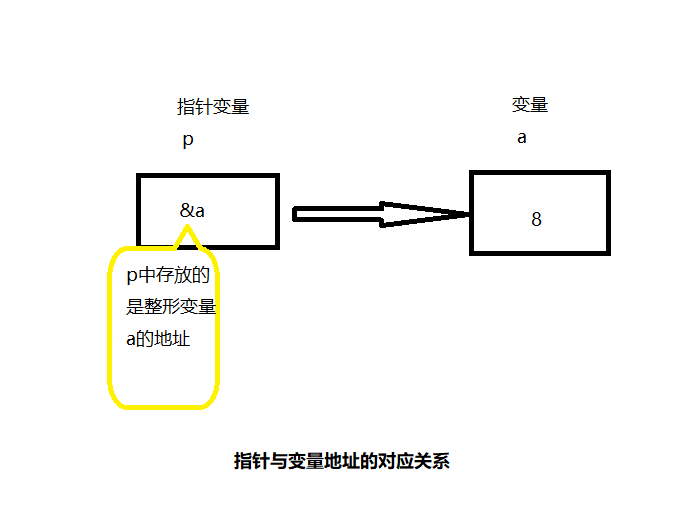2.指针变量的使用 两个重要运算符： （1）&：取地址运算符，如p=&a; 则p为变量a的地址。 （2）：指针运算符，后面只能接指针变量。用于访问指针变量所指向的变量。如：*p表示访问指针变量p所指向的变量的内容。
int a=8,b;
int *p;
p=&a;

对变量a有两种访问方式： （1）直接访问。b=a; （2）通过指针变量间接访问。b=*p;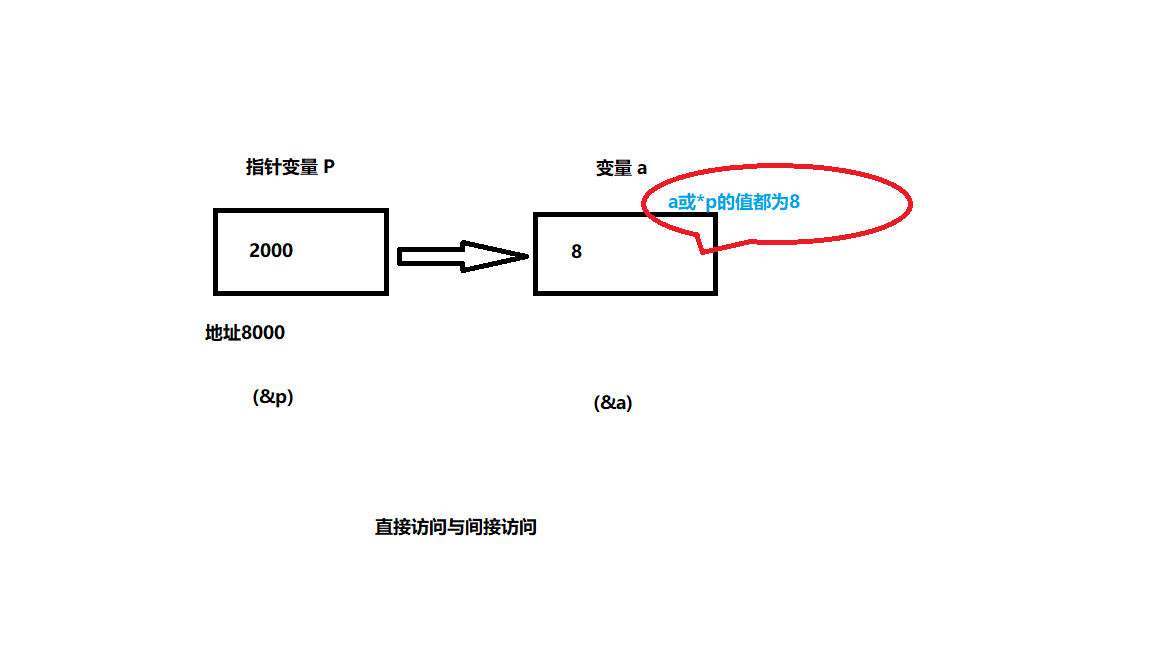变量a与指针变量p的引用
&a表示变量a的地址,&a=2000p指针变量p的值，p=2000=&a*p表示指针变量p指向的变量，*p=a=8&*p相当于&（*p）,&（*p）=&a=2000*&a相当于*（&a）,*（&a）=*p=a=8&p表示指针变量p的地址，&p=2000*&p相当于*（&p）,*（&p）=2000
注意：指针是一个地址，而指针变量是存放地址的变量。 3.指针的运算 （1）指针和整数的加减运算： 可以通过指针与整数的加减运算来移动指针p,实现对不同数据单元的访问操作。对不同的数据类型，移动的单位长度不同。单位长度一般是指针所指向的变量的数据类型长度。 格式一：p=p+n;(或p=p-n;) 格式二：p++;(或p–；) 格式三：++p;(或–p;) 注意： 指针变量的值加1（或减1），并不是给地址加1（或减1），而是加上（或减去）1个数据类型长度， 也就是指针所指向的变量在内存中所占的字节数。 （2）指针和指针的赋值运算：
int a=10,*p,*q;
p=&a;
q=p;
//p和q的值都是变量a的地址。

4.指向指针的指针 关系： （1）*二级指针变量：代表所指向的以及指针变量。如：*q就代表p; (2)**二级指针变量：代表它所指的一级指针变量所指向的变量。如：**q代表a; (3)*一级指针变量：代表它所指向的变量。如：*p代表a。

指针与数组

一。指向一维数组元素的指针
c语言数组中的数组元素可以看作相应类型的变量。只要类型匹配，就可以定义一个指针变量，让这个变量存储数组中数组元素的地址，则该指针便指向该数组元素。
int a = { 1, 2, 3, 4, 5, 6, 7, 8, 9, 10 };    //定义a为包含十个数据的数组
int *p; //p为指向整型变量的指针变量
p = &a; //把a元素的地址赋给指针变量p
//p=a;       与p = &a等效，p的值是数组a首元素的地址

通过指针引用一维数组元素： 1.指向一维数组首元素的指针 假设指针p指向一维数组a的第一个元素a。则： p+1: 使p指向下一个元素a. p+i： 使p指向元素a[i]. 注意!!! （1）可以使用*(p+i)访问元素a[i]。 （2）因为p和a都表示数组首地址，所以p+i也可以记作a+i,指向元素a[i]。 （3）指向数组的指针变量也可以带下标，如：p[i]与*(p+i)和*(a+i)等价,表示元素a[i]。 由此可知：当指针变量p指向一维数组a，即指向一维数组的第一个元素a后，数组的第i+1个元素有以下4种写法： a[i] ; p[i] ; *(a+i) ; *(p+i) 。 a[i]的地址也对应有4种写法： &a[i] ; &p[i] ;a+i ; p+i ; 2.指向一维数组非首元素的指针
例：
int a,*p;
p=&a;
//用法较少

举例： (1)利用指向数组元素的指针输出数组a的各个元素。

#include<stdio.h>
#include<stdlib.h>
int main()
{
int a = { 11,22,33,44,55,66 };
int *p;
int i;
for (p = a; p < a + 5; p++)
{
printf("%4d", *p);
i++;
if (i == 6)
break;  //避免指针越界
}
system("pause");
return 0;
}

（2）字符串复制：实现将字符数组str2中的字符串复制到字符数组str1中。
// 方法一：用数组名[下标]来访问数组元素
#include<stdio.h>
#include<stdlib.h>
int main()
{
int i;
char str1, str2 = { "how are you?" };
for (i = 0; (str1[i] = str2[i]) != '\0'; i++);
puts(str1);
system("pause");
return 0;
}

// 方法二：用指针名[下标]来访问数组元素
#include<stdio.h>
#include<stdlib.h>
int main()
{
int i;
char str1, str2 = { "how are you?" };
char *p1 = str1, *p2 = str2;
for (i = 0; (p1[i] = p2[i]) != 0; i++);
puts(str1);
system("pause");
return 0;
}

// 方法三：用指针名加偏移量计算出来的地址来访问数组元素
#include<stdio.h>
#include<stdlib.h>
int main()
{
int i;
char str1, str2 = { "how are you?" };
char *p1 = str1, *p2 = str2;
for (i = 0; (*(p1+i) = *(p2+i)) != 0; i++);
puts(str1);
system("pause");
return 0;
}

// 方法四：用数组名加偏移量计算出来的地址来访问数组元素
#include<stdio.h>
#include<stdlib.h>
int main()
{
int i;
char str1, str2 = { "how are you?" };
for (i = 0; (*(str1+i) = *(str2+i)) != 0; i++);
puts(str1);
system("pause");
return 0;
}

一维数组中几个关键符号的理解： 以int buf={0}为例： 1.buf：两层含义，一是数组名，sizeof(buf)时，就是数组名的含义。二是等价于&buf，表示数组第一个元素的首字节地址，是一个常量值。（不能作为左值（作为数组名时，不包括数组的初始化），作为右值时，表示一个地址） 2.buf：第一个元素的空间，可对其进行读写操作，作为左值被写，作为右值被读。 3.&buf：等价于buf，是一个地址常量，只能作为右值。 4.&buf：表示数组首地址，是一个地址常量，只能作为右值。 buf与&buf的值相等，但含义不同。printf("%p\n",buf)与printf("%p\n",&buf)打印结果相同，表示值相等。printf("%p\n",buf+1)与printf("%p\n",&buf+1)打印结果完全不相同。buf表示数组的第一个元素首字节的地址，加1加的是一个元素空间的大小；&buf表示数组首地址，加1加的是整个数组空间大小，主要用于构造多维数组。
二。指向二维数组元素的指针 当指针p指向二维数组中的某个元素后，可以用指针p访问二维数组的所有元素。 例：
int a;
int *p = &a;

p指向二维数组a的首元素，如图一。若将数组元素用指针p表示出来，如图二。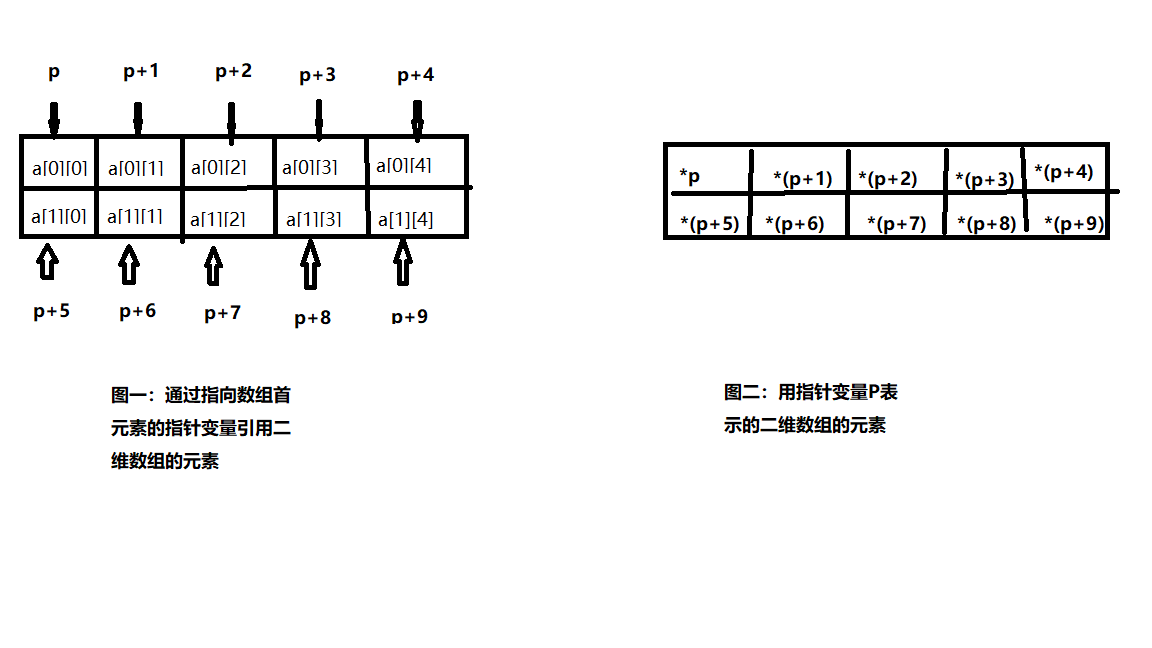假设指针变量p已经指向共有M行N列的数组A的首元素，则： A[i][j]的地址是：p+i * N+j ;A[i][j]可表示为：*(p+i * N+j)。 二维数组a[ ][ ]的有关指针 （注意：&a[i]和a[i]值虽然一样，但&a[i]或a+i指向行，而a[i]或 * (a+1)指向列）
表示形式含义a二维数组名，指向一维数组a, 即0行起始地址a,*(a+0),*a0行0列元素的地址a+1,&a1行起始地址a,*(a+1)1行0列元素a的地址a+2,*(a+1)+2,&a1行2列元素a的地址*(a+2), ((a+1)+2),a1行2列元素a的值
例：输出二维数组的有关数据（地址和元素的值）。
#include<stdio.h>
#include<stdlib.h>
int main()
{
int a = { 1, 3, 5, 7, 9, 11, 13, 15, 17, 19, 21, 23 };
printf("%d  %d\n\n", a, *a);		//0行起始地址  ，  0行0列元素的地址
printf("%d  %d\n\n", a, *(a + 0));  //0行0列起始地址
printf("%d  %d\n\n", &a, &a);  //0行起始地址 ，   0行0列元素的地址
printf("%d  %d\n\n",a,a+1);  //1行0列元素地址 ，   第1行起始地址
printf("%d  %d\n\n",&a,*(a+1)+0);  //1行0列元素地址
printf("%d  %d\n\n",a,*(a+2));  // 2行0列元素地址
printf("%d  %d\n\n",&a,a+2); //2行起始地址
printf("%d  %d\n\n",a,*(*(a+1)+0));   //1行0列元素的值
printf("%d  %d\n\n",*a,*(*(a + 2) + 0));  //2行0列元素的值
system("pause");
return 0;
}


运行结果：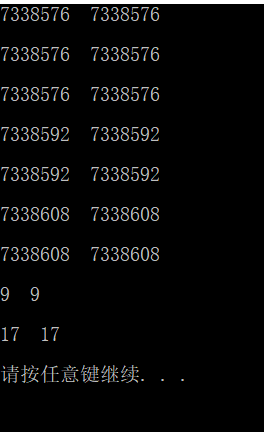例：用指针法求二维数组中的最大值。
#include<stdio.h>
#include<stdlib.h>
int main()
{
int a, max, i, j;
int *p = &a;
printf("请输入数组中的各元素\n");
for (i = 0; i < 2; i++)
{
for (j = 0; j < 5; j++)
{
scanf("%d", p++);		//通过p++依次引用各数组元素的地址
}
}
max = a;
p = &a;
for (i = 0; i < 2; i++)
{
for (j = 0; j < 5; j++)
{
if (max < *(p + i * 5 + j))
max = *(p + i * 5 + j);		//max总是存放比较大的数
}
}
for (i = 0; i < 2; i++)
{
for (j = 0; j < 5; j++)
{
printf("%5d", *(p + i * 5 + j));	//输出数组
}
}
printf("\n数组中的最大值为：%d\n", max);
system("pause");
return 0;
}

上述例子为指针指向整型变量，下面给出例子为：指针指向一个包含m个元素的一维数组。
//输出二维数组任一行任一列元素的值。
#include<stdio.h>
#include<stdlib.h>
int main()
{
int a = { 1, 3, 5, 7, 9, 11, 13, 15, 17, 19, 21, 23 };		//定义二维数组并初始化
int(*p), i, j;  //指针变量p是指向包含4个整型元素的一维数组
p = a;		//p指向二维数组的0行
printf("输入要求输出的行号和列号:\n");
scanf("%d%d", &i, &j);
printf("a[%d][%d]=%d\n", i, j, *(*(p + i) + j));		//输出a[i][j]的值
system("pause");
return 0;
}

三.指向数组首元素的指针变量的运算 若指针变量p指向数组a的首元素，则： （1）p++(或p+=1) p指向下一个元素。 （2）*p++ 相当于 *(p++)。都是先取 *p 的值，然后使p加1。 （3） *(p++)与 *(++p) *(p++)同 *p++，先取 *p 的值，然后使p加1； *(++p)先使p加1，然后取 *p 的值。 若p初值为a(即&a)，若输出 *(p++)，得到a的值，若输出 *(++p)，得到a的值。 （4）（*p）++ 表示p指向的元素值加1。相当于（a++）。 （5）如果指针指向数组a的非首元素a[i]。则：
*(p–)相当于a[i–]，先取 *p,再使p减1.*(p++)相当于a[++i],先使p+1,在取 *p.*(–p)相当于a[–i],先使p-1,在取 *p.
指针数组和数组指针的区别：
指针数组 指针数组：指针数组可以说成是”指针的数组”，首先这个变量是一个数组。 其次，”指针”修饰这个数组，意思是说这个数组的所有元素都是指针类型。 在 32 位系统中，指针占四个字节。 数组指针 数组指针：数组指针可以说成是”数组的指针”，首先这个变量是一个指针。 其次，”数组”修饰这个指针，意思是说这个指针存放着一个数组的首地址，或者说这个指针指向一个数组的首地址。
指针数组的例题：
//将若干字符串按字母顺序（由小到大）输出
#include<stdio.h>
#include<stdlib.h>
#include<string.h>
void sort(char *name[], int n)		//对字符串排序
{
char *t;
int i, j, k;
for (i = 0; i < n - 1; i++)		//	用选择法排序
{
k = i;
for (j = i+1; j < n; j++)
{
if (strcmp(name[k], name[j])>0)
k = j;
}
if (k != i)
{
t = name[i];
name[i] = name[k];
name[k] = t;
}
}
}
void print(char *name[], int n)		//打印
{
int i;
for (i = 0; i < n; i++)
{
printf("%s\n", name[i]);
}
}
int main()
{
char *name[] = { "Follow me", "BASIC", "Great Wall", "FOREVER", "Computer design" };		//指针数组的值为各字符串的首字符的地址
int n = 5;
sort(name, n);		//实参为数组首元素的地址，形参为指针数组名
print(name, n);
system("pause");
return 0;
}


*指针的一些复杂说明：
int p; 这是一个普通的整型变量int *p 首先从 p 处开始,先与*结合,所以说明 p 是一个指针, 然后再与 int 结合, 说明指针所指向的内容的类型为 int 型。所以 p 是一个返回整型数据的指针。int p – 首先从 p 处开始,先与[] 结合,说明 p 是一个数组, 然后与 int 结合, 说明数组里的元素是整型的, 所以 p 是一个由整型数据组成的数组。int *p首先从 p 处开始, 先与 [] 结合, 因为其优先级比 * 高,所以 p 是一个数组, 然后再与 * 结合, 说明数组里的元素是指针类型, 然后再与 int 结合, 说明指针所指向的内容的类型是整型的, 所以 p 是一个由返回整型数据的指针所组成的数组。(指针数组)int (*p) 首先从 p 处开始, 先与 * 结合,说明 p 是一个指针然后再与 [] 结合(与"()"这步可以忽略,只是为了改变优先级), 说明指针所指向的内容是一个数组, 然后再与int 结合, 说明数组里的元素是整型的。所以 p 是一个指向由整型数据组成的数组的指针。(数组指针)int **p 首先从 p 开始, 先与 * 结合, 说是 p 是一个指针, 然后再与 * 结合, 说明指针所指向的元素是指针, 然后再与 int 结合, 说明该指针所指向的元素是整型数据。由于二级指针以及更高级的指针极少用在复杂的类型中, 所以后面更复杂的类型我们就不考虑多级指针了, 最多只考虑一级指针。int p(int) 从 p 处起,先与 () 结合, 说明 p 是一个函数, 然后进入 () 里分析, 说明该函数有一个整型变量的参数, 然后再与外面的 int 结合, 说明函数的返回值是一个整型数据。int (*p)(int)从 p 处开始, 先与指针结合, 说明 p 是一个指针, 然后与()结合, 说明指针指向的是一个函数, 然后再与()里的 int 结合, 说明函数有一个int 型的参数, 再与最外层的 int 结合, 说明函数的返回类型是整型, 所以 p 是一个指向有一个整型参数且返回类型为整型的函数的指针。int *(*p(int)) 可以先跳过, 不看这个类型, 过于复杂从 p 开始,先与 () 结合, 说明 p 是一个函数, 然后进入 () 里面, 与 int 结合, 说明函数有一个整型变量参数, 然后再与外面的 * 结合, 说明函数返回的是一个指针, 然后到最外面一层, 先与[]结合, 说明返回的指针指向的是一个数组, 然后再与 * 结合, 说明数组里的元素是指针, 然后再与 int 结合, 说明指针指向的内容是整型数据。所以 p 是一个参数为一个整数据且返回一个指向由整型指针变量组成的数组的指针变量的函数。

指针与函数

一。指针做函数参数 指针可以作为参数在调用函数和被调用函数之间传递数据，传递的是“地址值”。
如果有一个实参数组，要想改变此数组中元素的值，实参与形参的对应关系有四种：
形参和实参都用数组名。（不举例）实参用数组名，形参用指针变量。实参为指针变量，形参用数组名。形参和实参都用指针变量。（实参中指针指向数组）
1.函数形参为指针，实参为地址表达式 例：利用指针做参数，交换两个变量的值。
#include<stdio.h>
#include<stdlib.h>
void swap1(int *a, int *b)
{
int c;
c = *a;	//*为解引用操作
*a = *b;
*b = c;
}

int main()
{
int a = 10, b = 100;
swap1(&a, &b);
printf("调用swap1函数后，主函数中a和b的值为%d  %d\n", a, b);
system("pause");
return 0;
}

执行结果为：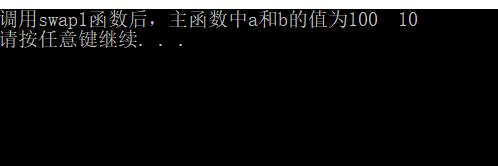#include<stdio.h>
#include<stdlib.h>
void swap2(int *a, int *b)
{
int *c;
c = a;
a = b;
b = c;
}
int main()
{
int a = 10, b = 100;
swap2(&a, &b);
printf("调用swap2函数后，主函数中a和b的值为%d  %d\n", a, b);
system("pause");
return 0;
}

执行结果为：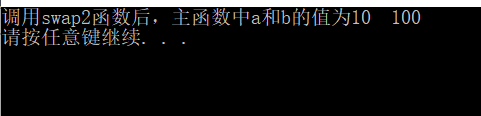分析： 很明显，swap1函数正确，两个变量值得到了交换，而swap2函数调用后两个值并没有交换。 因为swap2函数虽然经过了一系列的变换，但是地址中的内容没有变。
不能企图通过改变指针形参的值而使指针实参的值而改变。
2.函数形参为指针，实参为数组名 例：将数组a中的n个整数按相反顺序存放。
#include<stdio.h>
#include<stdlib.h>
void fun(int *x, int n)		//形参为指针
{
int *p, *i, *j;
int temp,m;
m = (n - 1) / 2;
j = x + n - 1;
p = x + m;  //p指向a[m]的地址
for (i = x; i <= p; i++, j--)
{
temp = *i;  //交换a[i]和a[j]
*i = *j;
*j = temp;
}
}
int main()
{
int i, a = { 1, 2, 3, 4, 5, 6, 7, 8, 9, 10 };
printf("原顺序为：\n");
for (i = 0; i < 10; i++)
{
printf("%4d", a[i]);
}
printf("\n");
fun(a, 10);		//实参为数组名
printf("排序后为：\n");
for (i = 0; i < 10; i++)
{
printf("%4d", a[i]);
}
printf("\n");
system("pause");
return 0;
}

运行结果为：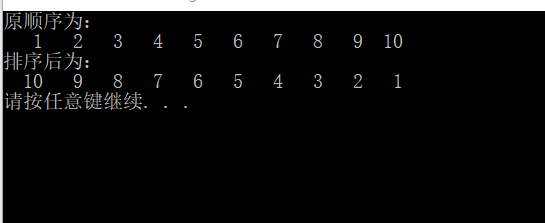用指针做形参，数组名做实参，有两种方法：用指向变量的指针变量用指向一维数组的指针变量。举例如下：
//1.有3个学生，各学4门课，计算总平均成绩，第n个学生的学习成绩
#include<stdio.h>
#include<stdlib.h>
void average(float *p, int n)     //求平均成绩的函数
{
float *p1;
float sum = 0, aver;
p1 = p + n - 1;		//p1指向最后一个元素
for (p; p <= p1; p++)		//使p先后指向二维数组的各个元素
{
sum = sum + (*p);
}
aver = sum / n;
printf("总平均成绩为：%.2f\n", aver);
}

void search(float(*p), int n)     //p是指向具有四个元素的一维数组的指针
{
int i;
for (i = 0; i < 4; i++)
{
printf("%.2f  ", *(*(p + n) + i));
}
printf("\n");
}
int main()
{
int x;
float score = { { 65, 67, 70, 60 }, { 80, 87, 90, 81 }, { 90, 99, 100, 98 } };
average(*score, 12);  //12个成绩的平均分。  实参： *score=score=&score

scanf("%d", &x);
printf("输出第%d个学生的成绩:\n", x);
search(score, x);		//实参：score代表该数组第0行起始地址
system("pause");
return 0;
}

运行结果为：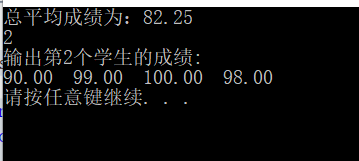//2.在题1的基础上，查找有一门以上课程不及格的学生，输出他们全部课程的成绩。
#include<stdio.h>
#include<stdlib.h>
void search(float(*p), int n)		 //形参：指针p指向包含4个元素的一维数组
{
int i, j, flag;
for (j = 0; j < n; j++)
{
flag = 0;
for (i = 0; i < 4; i++)
{
if (*(*(p + j) + i) < 60)		//p+j为score数组第j行的起始地址，*(p + j)=&score[j]  ，*(p + j)+i=&score[j][i]。
flag = 1;
}
if (flag == 1)
{
printf("第%d个学生不及格，他的成绩为：\n", j + 1);
for (i = 0; i < 4; i++)
{
printf("%.2f  ", *(*(p + j) + i));		//*(*(p + j) + i)就是score[j][i]的值
}
printf("\n");
}
}
}
int main()
{
float score = { { 65, 57, 70, 60 }, { 50, 87, 90, 81 }, { 90, 99, 100, 98 } };
search(score, 3);
system("pause");
return 0;
}

结果为：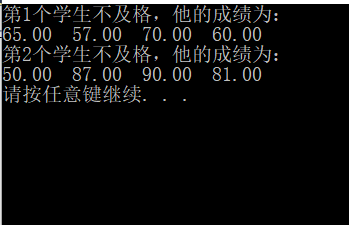3.实参为指针变量，形参用数组名。 举例：
//对10个整数由从大到小排序
#include<stdio.h>
#include<stdlib.h>
void fun(int x[], int n)		//形参为数组名
{
int i, j, k, t;
for (i = 0; i < n - 1; i++)
{
k = i;				    //选择排序
for (j = i + 1; j < n; j++)
{
if (x[j]>x[k])
k = j;
}
if (k != i)
{
t = x[i];
x[i] = x[k];
x[k] = t;
}
}
}
int main()
{
int i, a, *p;
p = a;
printf("请输入10个数\n");
for (i = 0; i < 10; i++)
{
scanf("%4d", p++);
}
p = a;				//很重要！！使指针重新指向a
fun(p, 10);		//实参为指针
printf("排序后的数为：\n");
for (p = a, i = 0; i < 10; i++)
{
printf("%4d", *p);
p++;
}
printf("\n");
system("pause");
return 0;
}

4.实参和形参都为指针变量 举例：
//对10个整数由从大到小排序
#include<stdio.h>
#include<stdlib.h>
void fun(int *x, int n)		//形参为指针
{
int i,j,k,t;
for (i = 0; i <n-1; i++)
{
k = i;				    //选择排序
for (j = i + 1; j < n; j++)
{
if (*(x+j)>*(x+k))
k = j;
}
if (k != i)
{
t = *(x+i);
*(x + i) = *(x + k);
*(x + k) = t;
}
}
}
int main()
{
int i, a, *p;
p = a;
printf("请输入10个数\n");
for (i = 0; i < 10; i++)
{
scanf("%4d", p++);
}
p = a;				//很重要！！使指针重新指向a
fun(p, 10);		//实参为指针
printf("排序后的数为：\n");
for (p = a, i = 0; i < 10; i++)
{
printf("%4d", *p);
p++;
}
printf("\n");
system("pause");
return 0;
}

函数指针与指针函数
一。函数指针：
1.定义：
函数指针是指向函数的指针变量，本质上是一个指针变量，表示的是一个指针，它指向的是一个函数，其形式一般如下：
类型说明符 （*函数名）（参数）
例如，int(*p)(int ，int)； 定义p是一个指针，指向函数类型为整型且有两个整型参数的函数。 此时指针p的类型用：int( * )(int ,int)来表示。 2.用函数指针调用函数 （1）通过函数名调用函数。
//输出a和b中的最大值。
#include<stdio.h>
#include<stdlib.h>
int max(int x, int y)
{
return((x > y) ? x : y);
}
int main()
{
int a, b, c;
printf("输入a和b的值\n");
scanf("%d%d", &a, &b);
c = max(a, b);
printf("最大值为：%d", c);
system("pause");
return 0;
}

（2）通过指针调用它所指向的函数
//输出a和b中的最大值。
#include<stdio.h>
#include<stdlib.h>
int max(int x, int y)
{
return((x > y) ? x : y);
}
int main()
{
int(*p)(int, int);		//定义函数指针
p = max;
int a, b, c;
printf("输入a和b的值\n");
scanf("%d%d", &a, &b);
c =(*p)(a, b);
printf("最大值为：%d", c);
system("pause");
return 0;
}

3。用函数指针做函数参数
原理简述：
有一个函数（fun），有两个形参（x1和x2），定义x1和x2为指向函数的指针。在调用函数fun时，实参为两个函数名f1，f2，给形参传递的是函数f1和f2的入口地址，这样在fun中就可以调用f1和f2函数了。
void fun(int(*x1)(int), int(*x2)(int, int))	    //形参为函数指针（f1,f2）
{
int a, b, i = 3,j = 5;
a = (*x1)(i);			//调用f1函数，i是形参
b = (*x2)(i, j);		//调用f1，f2函数，i,j是形参
}

例题： 有两个整数a和b，由用户输入1，2，3其中一个值。如输入1，则程序给出最大值，如输入2，则给出最小值，如输入3，则求两数之和。
#include<stdio.h>
#include<stdlib.h>
int fun(int x, int y, int(*p)(int, int))			//形参为函数指针
{
int result;
result = (*p)(x, y);
printf("%d\n", result);		//输出结果
return(result);
}
int max(int x, int y)
{
printf("最大值为：\n");
return((x > y) ? x : y);
}
int min(int x, int y)
{
printf("最小值为：\n");
return((x < y) ? x : y);
}
{
int z;
z = x + y;
printf("和为：\n");
return (z);
}
int main()
{
int a = 100, b = -10,n;
printf("请输入1或2或3：\n");
scanf("%d", &n);
if (n == 1)
fun(a, b, max);
else if (n == 2)
fun(a, b, min);
else if (n == 3)
else
printf("请重新输入。");
system("pause");
return 0;
}


二。指针函数
1.定义： 是指带指针的函数，本质上是一个函数，函数返回类型是某一类型的指针， 其形式一般如下所示：
类型标识符 *函数名（参数列表）
例如： int *max(int x,int y); 函数名为max，其返回值为指针，指向整型数据。
例题：
//1.有a个学生，每个学生有b门课程的成绩。要求用户输入学号后，输出该学生的全部成绩。
//用指针函数来实现。

#include<stdio.h>
#include<stdlib.h>
float *search(float(*pointer), int n)		//search为指针函数，  形参pointer是指向一维数组的指针变量
{
float *pt;
pt = *(pointer + n);		//pt的值为：&score[k]
return(pt);
}
int main()
{
float score[] = { { 65, 57, 70, 60 }, { 50, 87, 90, 81 }, { 90, 99, 100, 98 } };
float *p;
int i, k;
printf("输入你要找的学生学号：\n");
scanf("%d", &k);
printf("学生%d的成绩为：\n", k);
p = search(score, k-1);  //实参为数组首行地址， 返回score[k]的地址
for (i = 0; i < 4; i++)
{
printf("%.2f\t", *(p + i));		//输出score[k]——score[k]的值。
}
printf("\n");
system("pause");
return 0;
}

结果为：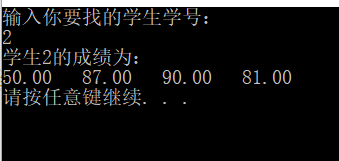//在第一题的基础上，找出有不及格的成绩的学生和学号。
#include<stdio.h>
#include<stdlib.h>
float *search(float(*pointer))		//search为指针函数，  形参pointer是指向一维数组的指针变量
{
int i;
float *pt;
pt =NULL;		//初始化
for (i = 0; i < 4; i++)
{
if (*(*pointer + i) < 60)
pt = *pointer;				//有不及格，使pt指向score[i]
}
return(pt);
}
int main()
{
float score[] = { { 65, 57, 70, 60 }, { 50, 87, 90, 81 }, { 90, 99, 100, 98 } };
float *p;
int i, j;
for (i = 0; i < 3; i++)
{
p = search(score + i);	//调用search函数，如果有不及格则返回score[i]的地址，否则返回NULL.
if (p == *(score + i))		//如果返回score[i]的地址，则p不为空。
{
printf("学生%d的成绩为：\n", i+1);
for (j = 0; j < 4; j++)
{
printf("%.2f\t", *(p + j));		//输出score[i]——score[i]的值。
}
printf("\n");
}
}
printf("\n");
system("pause");
return 0;
}

结果为：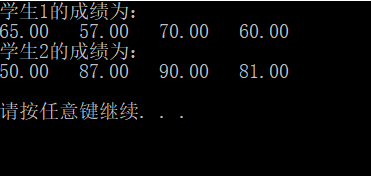指针与字符串

1.使指针变量指向字符串的方法 （1）在定义的同时给指针变量赋初值。 char *p=“Gold medal”; （2）给指针变量赋值。 char *p; p=“Gold medal”;
#include<stdio.h>
#include<stdlib.h>
int main()
{
char *p = "Gold medal";
printf("%s", p);
system("pause");
return 0;
}


#include<stdio.h>
#include<stdlib.h>
int main()
{
char *p = "Gold medal";
while (*p != '\0')
{
printf("%c", *p++);
}
system("pause");
return 0;
}


都是将字符串的第一个字符的地址赋给指针变量p。 C语言对字符串常量是按字符数组处理的，在内存中开辟一个字符数组来存放字符串常量（包括字符串结束符‘\0’），程序在定义字符指针变量p时只是把字符串的首地址赋给p,而不能把整个字符串赋给p。 2.指向字符串常量的指针变量的使用 （1）把字符串当作整体来处理
scanf("%s",指针变量);gets(指针变量)；printf("%s",指针变量)；puts(指针变量)；
（2）处理字符串中的单个字符 若指针已经指向了字符串常量，则用指针变量表示的第i个字符为： *（指针变量+i） 3.字符串中字符的存取方式
下标方法指针方法 例题： 将字符串a复制为字符串b，然后输出字符串b。（两种方法）
//1。数组的方法
#include<stdio.h>
#include<stdlib.h>
int main()
{
char a[] = "I am a student.", b;  //定义字符数组
int i;
for (i = 0; *(a + i) != '\0'; i++)
{
*(b + i) = *(a + i);		//将a[i]的值赋给b[i]
}
*(b + i) = '\0';		//数组b的有效字符后加'\0'
printf("字符串a是：%s\n", a);
printf("字符串b是：\n");
for (i = 0; b[i] != '\0'; i++)
{
printf("%c", b[i]);		//逐个输出字符
}
printf("\n");
system("pause");
return 0;
}

//2。指针的方法
#include<stdio.h>
#include<stdlib.h>
int main()
{
char a[] = "I am a student.", b;  //定义字符数组
char *p1, *p2;
p1 = a; p2 = b;		//指针分别指向数组的第一个元素
for (p1; *p1 != '\0'; p1++,p2++)
{
*p2 = *p1;		//将p1所指向的元素的值赋给p2所指向的元素
}
*p2 = '\0';		//有效字符后加'\0'
printf("字符串a是：%s\n", a);
printf("字符串b是：%s\n", b);
system("pause");
return 0;
}

4.使用字符指针变量与字符数组的区别 （1）存储内容不同： 字符指针变量本身是一个变量，用于存放字符串的首地址，编译时只为其分配一个存储单元。而字符串本身就是存放在以该首地址为首的一块连续内存空间中并以‘\0’作为结束标志。 字符数组是由若干数组元素组成，每个元素中放一个字符,编译时为其分配若干存储单元。 （2）赋值方法不同： 对于字符指针变量，可用以下方法赋值： char *p; p=“Gold medal”; 而字符数组只能对各个元素逐个赋值，不能对数组名赋值。
char p;
p = "Gold medal";
//该种赋值方法错误

（3）指针变量的值是可以改变的。 而数组名虽是地址，但它的值是不能改变的。
#include<stdio.h>
#include<stdlib.h>
int main()
{
char *p ="Gold medal";
p = p + 5;
printf("%s", p);
system("pause");
return 0;
}
//运行结果为：
medal

char p[] ="Gold medal";
p = p + 5;
printf("%s", p);
//是错误的

（4）输入字符串时有区别； 对于字符指针变量：
	char *p;
scanf("%s", p);
//是不可以的。但是可以这样输入：

char str;
char *p=str;  //使p指向了确定内存
scanf("%s", p);

对于字符数组：
char p;
scanf("%s", p);
//是可以的

5.字符指针做函数参数 在被调函数中可以改变字符串的内容，在主调函数中可以引用改变后的字符串。 （1）用字符数组名作为函数参数。
//用函数调用实现字符串的复制。
#include<stdio.h>
#include<stdlib.h>
void copy(char from[], char to[])		//形参为字符数组
{
int i = 0;
while (from[i] != '\0')
{
to[i] = from[i];
i++;
}
to[i] = '\0';
}
int main()
{
char a[] = "I am a student.";
char b[] = "You are a teacher.";
printf("原字符串a为：%s\n", a);
printf("原字符串b为：%s\n", b);
printf("把字符串a复制到字符串b中\n");
copy(a, b);
printf("复制后的字符串为：\n%s\n%s\n", a, b);
system("pause");
return 0;
}

（2）用字符型指针做为函数实参
#include<stdio.h>
#include<stdlib.h>
void copy(char from[], char to[])		//形参为字符数组
{
int i = 0;
while (from[i] != '\0')
{
to[i] = from[i];
i++;
}
to[i] = '\0';
}
int main()
{
char a[] = "I am a student.";
char b[] = "You are a teacher.";
char *from = a, *to = b;
printf("原字符串a为：%s\n", a);
printf("原字符串b为：%s\n", b);
printf("把字符串a复制到字符串b中\n");
copy(from, to);		//实参为字符指针变量
printf("复制后的字符串为：\n%s\n%s\n", a, b);
system("pause");
return 0;
}

（3）用字符型指针做为函数实参和形参
#include<stdio.h>
#include<stdlib.h>
void copy(char *from, char *to)		//形参为字符指针变量
{
int i = 0;
for (from; *from != '\0';from++,to++)
{
*to = *from;
}
*to = '\0';
}
int main()
{
char *a = "I am a student.";
char b[] = "You are a teacher.";
char *p=b;
printf("原字符串a为：%s\n", a);
printf("原字符串b为：%s\n", b);
printf("把字符串a复制到字符串b中\n");
copy(a,p);		//实参为字符指针变量
printf("复制后的字符串为：\n%s\n%s\n", a, b);
system("pause");
return 0;
}

此篇博客创作属实不易，博主熬到半夜好几天啦，一字一句地可算整理出来了。如果有错误，请多多指教。
展开全文• C++指针1、什么是指针2、指针定义和赋值3、引用指针变量4、指针作函数参数5、字符串与指针6、函数与指针7、指向指针指针8、const指针相关【难点】9、void类型指针10、有关指针数据类型的小结 1、什么是指针 如果把...C++ C++指针 C语言
• c语言指针的基础知识点都在这里，可以帮助你更好的了解指针。
• 指针C语言中的难点，也是C语言中的精华所在，本文档列举了指针使用中的常见注意事项及易混淆知识点的对比，为C语言的初学者掌握指针提供了清晰的思路。
• 关于指针总结归纳 1.指针的含义：指针即是地址 2.指针的定义： `变量类型 变量名 int a 函数类型 函数名 int a（）； 数组类型 数组名 int a[]; 类比可得 指针类型 指针变量名 int * a； ...
• 这个总结尚未包含C语言数据结构与算法部分，后续会陆续更新出来，文中有漏掉的知识点，还请大家多多指正。　 总体上必须清楚的: 　1)程序结构是三种:顺序结构、选择结构(分支结构)、循环结构。 　2)读程序都要从...
• 总结指针C语言的灵魂 ★指针的定义 ☆地址 内存单元的编号 从零开始的非负整数 范围：4G ☆指针 1.指针就是地址，地址就是指针 2.指针变量是存放地址的变量 3.指针指针变量是两个不同的概念 4.叙述时通常把...
• 怎么做好嵌入式？相信这个问题无论问谁你都会得到一句学好C语言！今天推荐一篇大佬写的嵌入式C语言知识点总结，非常值得一读。从语法上来说C语言并不复杂， 但编写优质可靠的嵌入式C程序并非易事...嵌入式 编程语言 java linux python
• 这篇C语言指针学习经验总结主要是我入职以来学习C指针过程中的点滴记录。文档里面就不重复书上说得很清楚的概念性东西，只把一些说得不清楚或理解起来比较费解的东西做一下讲解，希望能达到以下三个目的 1.通过写...
• 但是，指针又是c语言中极其重要的知识点，可以说是一个坎，跨过这个坎，对于C语言的理解就会更上一层楼！ 二、基础知识 1.什么是指针、引用和取值 指针是一个存储计算机内存地址的变量，即指针变量里面存放的是...
• ## c语言指针的有关总结

万次阅读 多人点赞 2020-09-07 22:18:51
指针指针的概念1：对内存的理解2：地址(重点)指针定义与指针有关的运用1：c语言动态内存管理 内存泄漏？ 指针的概念 1：对内存的理解 这是我们计算机的内存条。这当然是我们的物理内存 这是我们的虚拟内存 像我们...内存管理
• 总结： 总的来说，就是带头结点时不管是否为空表，头指针的值都不会变化，都指向头结点。而不带头结点则需要根据不同情况来修改头指针的值。所以操作不统一，有所不便，所以绝大数时候使用带头结点的方式较为方便。...
• C语言最重要的知识点 总体上必须清楚的: 1)程序结构是三种: 顺序结构 、选择结构(分支结构)、循环结构。 其实做为一个开发者，有一个学习的氛围跟一个交流圈子特别重要这里我推荐一个C语言C++交流群583650410，不管...c++ 编程语言
• 在了解指针之前，首先要了解地址，我们平时的每一个变量，都是被储存在内存中的，每个内存又有一个编号，这个编号就被称为地址，如果把学校的宿舍楼理解成电脑的内存，整型变量理解为男生宿舍，把浮点型变量理解为...
• 指针运算符 指针运算符：& 和 * 结合方向：右 → 左 运算符与含义 对象个数 优先级 &（取地址运算符） 1 单目运算符 2 *（指针运算符） 1 单目运算符 2 【1】取地址运算符：& &a 是变量a的地址 【2】指针运算符（或...
• ## C语言基础知识梳理总结

万次阅读 多人点赞 2017-03-06 09:54:48
C语言是当代人学习及生活中的必备基础知识，应用十分广泛，下面为大家带来C语言基础知识梳理总结C语言零基础入门绝对不是天方夜谭！
• ## C语言指针相关知识理解以及总结

千次阅读 多人点赞 2017-07-17 09:05:31
C语言指针相关知识理解以及总结，什么是指针，关于*和&，指针变量运算，C语言数组指针（指向数组的指针），C语言字符串指针，二级指针，C语言指针数组，C语言与二维数组，函数指针，指针总结内存 liunx
• 总结 3.3 内存对齐 Char 偏移量必须为sizeof(char)即1的倍数 int 偏移量必须为sizeof(int)即4的倍数 float 偏移量必须为sizeof(float)即4的倍数 double 偏移量必须为sizeof(double)即8的倍数 Short ...
• ## C语言知识点总结（完美版）

千次阅读 多人点赞 2020-02-02 21:24:24
C语言最重要的知识点 总体上必须清楚的: 1)程序结构是三种: 顺序结构 、选择结构(分支结构)、循环结构。 2)读程序都要从main()入口, 然后从最上面顺序往下读(碰到循环做循环,碰到选择做选择)，有且只有一个main...
• ## C语言知识点总结

万次阅读 多人点赞 2018-08-24 08:09:48
C语言最重要的知识点 总体上必须清楚的: 1)程序结构是三种: 顺序结构 、选择结构(分支结构)、循环结构。 2)读程序都要从main()入口, 然后从最上面顺序往下读(碰到循环做循环,碰到选择做选择)，有且只有一个main...c
• ## 大学C语言考试易错知识点总结

千次阅读 多人点赞 2019-12-28 23:02:17
文章目录运算符相关自增（减）前后缀逻辑运算符的短路问题赋值运算符的返回值问题运算符优先级的问题函数相关函数声明问题mian函数参数问题变量相关用字符...现在不少大学都把C语言作为一门必学的编程语言。而每到这...
• C语言最重要的知识点总结 1.C 语言的基础知识 2.数据输入、输出 3.表达式 4.三种循环结构 5.函数 6.指针 7.数组 8.考试、面试及日常工作中常用考点
• 在结构体或类中, 指针访问其成员函数或变量通过 “->” 运算符或者看代码注释部分, 注释部分的操作不推荐: #include #include using namespace std; struct STRUCT { string hello; }; int main() { STRUCT *......

# c语言指针知识点总结c语言 订阅